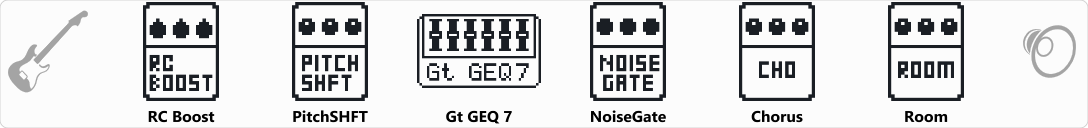# Bass Guitar Emulator

Discussion in 'Zoom G3n/G3Xn/G5n' started by paracelx, Jul 1, 2019.

1. Bass Guitar EmulatorDevice: Zoom G3n
Firmware: 2.20

Name on device: DG BASS
Optimized for: Guitar Amp

Effects chain:Made this to make my regular electric emulate a bass guitar. Let me know if you get it any better.

Effect: "RC Boost" (Overdrive / Distortion), active - "yes"
"Gain" = 77
"Bass" = 68
"Treble" = 39
"Volume" = 29

Effect: "PitchSHFT" (Modulation), active - "yes"
"Shift" = -12
"Fine" = 0
"Tone" = 0
"Balance" = 71

Effect: "Gt GEQ 7" (Filter), active - "yes"
"100 Hz" = 12.0
"200 Hz" = 3.0
"400 Hz" = -5.5
"800 Hz" = -12.0
"1.6 kHz" = -12.0
"3.2 kHz" = -12.0
"6.4 kHz" = 2.0
"Volume" = 80
"100 Hz" = 0.0
"200 Hz" = 0.0
"400 Hz" = 0.0
"800 Hz" = 0.0
"1.6 kHz" = 0.0
"3.2 kHz" = 0.0
"6.4 kHz" = 0.0
"Volume" = 80
"Parameter set" = A

Effect: "NoiseGate" (Dynamics), active - "yes"
"Detect" = EFXIN
"Depth" = 70
"Threshold" = 54
"Decay" = 19

Effect: "Chorus" (Modulation), active - "yes"
"Depth" = 33
"Rate" = 23
"Tone" = 7
"Mix" = 50

Effect: "Room" (Reverb), active - "yes"
"PreD" = 100
"Decay" = 18
"Mix" = 43
"Tail" = On

Patch Volume: 100